# ICSE Class 10 Mathematics 2019 Question Paper

Attempt all questions from Section A and any four questions from Section B.

### Section A (40 Marks)

Attempt all questions from this Section.

Question 1

(a) Solve the following inequation and write down the solution set:
11𝑥 − 4 < 15𝑥 + 4 ≤ 13𝑥 + 14, 𝑥𝜖𝑊
Represent the solution on a real number line. 

(b) A man invests Rs. 4500 in shares of a company which is paying 7.5% dividend. If Rs. 100 shares are available at a discount of 10%. Find:

1. Number of shares he purchases.
2. His annual income. 

(c) In a class of 40 students, marks obtained by the students in a class test (out of 10) are given below: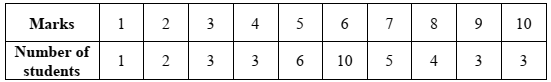Calculate the following for the given distribution:

1. Median
2. Mode 

Question 2

(a) Using the factor theorem, show that (x – 2) is a factor of 𝑥3 + 𝑥2 − 4𝑥 − 4. Hence factorise the polynomial completely. 

(b) Prove that:

(cosec 𝜃 − sin 𝜃)(sec 𝜃 − cos 𝜃)(tan 𝜃 + cot 𝜃) = 1 

(c) In an Arithmetic Progression (A.P.) the fourth and sixth terms are 8 and 14 respectively. Find the:

1. first term
2. common difference
3. sum of the first 20 terms. 

Question 3

(a) Simplify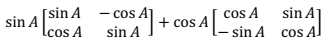(b) M and N are two points on the X axis and Y axis respectively. P(3, 2) divides the line segment MN in the ratio 2 : 3. Find:

1. the coordinates of M and N
2. slope of the line MN. 

(c) A solid metallic sphere of radius 6 cm is melted and made into a solid cylinder of height 32 cm. Find the:

2. curved surface area of the cylinder 

Take π = 3.1

Question 4

(a) The following numbers, K + 3, K + 2, 3K – 7 and 2K – 3 are in proportion. Find K. 

(b) Solve for x the quadratic equation 𝑥2 − 4𝑥 − 8 = 0. Give your answer correct to three significant figures. 

(c) Use ruler and compass only for answering this question.

Draw a circle of radius 4 cm. Mark the centre as O. Mark a point P outside the circle at a distance of 7 cm from the centre. Construct two tangents to the circle from the external point P.

Measure and write down the length of any one tangent. 

### Section B (40 Marks)

Attempt any four questions from this Section

Question 5

(a) There are 25 discs numbered 1 to 25. They are put in a closed box and shaken thoroughly. A disc is drawn at random from the box. Find the probability that the number on the disc is:

1. an odd number
2. divisible by 2 and 3 both.
3. a number less than 16. 

(b) Rekha opened a recurring deposit account for 20 months. The rate of interest is 9% per annum and Rekha receives Rs. 441 as interest at the time of maturity. Find the amount Rekha deposited each month. 

(c) Use a graph sheet for this question. Take 1 cm = 1 unit along both x and y axis.

1. Plot the following points: A(0,5), B(3,0), C(1,0) and D(1,–5)
2. Reflect the points B, C and D on the y axis and name them as B', C' and D' respectively.
3. Write down the coordinates of B', C' and D'.
4. Join the points A, B, C, D, D', C', B', A in order and give a name to the closed figure ABCDD'C'B'. 

Question 6

(a) In the given figure, ∠PQR = ∠PST = 90°, PQ = 5 cm and PS = 2 cm.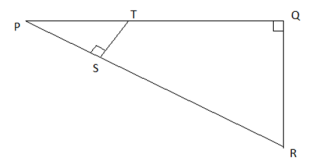1. Prove that ΔPQR ~ ΔPST.
2. Find Area of ΔPQR : Area of quadrilateral SRQT. 

(b) The first and last term of a Geometrical Progression (G.P.) are 3 and 96 respectively. If the common ratio is 2, find:

1. ‘n’ the number of terms of the G.P.
2. Sum of the n terms. 

(c) A hemispherical and a conical hole is scooped out of a solid wooden cylinder. Find the volume of the remaining solid where the measurements are as follows: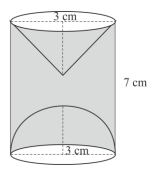The height of the solid cylinder is 7 cm, radius of each of hemisphere, cone and cylinder is 3 cm. Height of cone is 3 cm.

Give your answer correct to the nearest whole number. Take π = 22/7. 

Question 7

(a) In the given figure AC is a tangent to the circle with centre O. If ∠ADB = 55°, find x and y. Give reasons for your answers.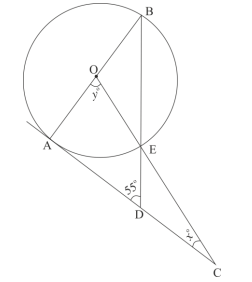(b) The model of a building is constructed with the scale factor 1 : 30.

1. If the height of the model is 80 cm, find the actual height of the building in meters.
2. If the actual volume of a tank at the top of the building is 27 m3, find the volume of the tank on the top of the model. 

(c) Given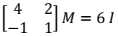where M is a matrix and I is unit matrix of order 2x2.

1. State the order of matrix M.
2. Find the matrix M. 

Question 8

(a) The sum of the first three terms of an Arithmetic Progression (A.P.) is 42 and the product of the first and third term is 52. Find the first term and the common difference. 

(b) The vertices of a ΔABC are A(3, 8), B(–1, 2) and C(6, –6). Find:

1. Slope of BC.
2. Equation of a line perpendicular to BC and passing through A. 

(c) Using ruler and a compass only construct a semi-circle with diameter BC = 7 cm. Locate a point A on the circumference of the semicircle such that A is equidistant from B and C. Complete the cyclic quadrilateral ABCD, such that D is equidistant from AB and BC. Measure ∠ADC and write it down. 

Question 9

(a) The data on the number of patients attending a hospital in a month are given below. Find the average (mean) number of patients attending the hospital in a month by using the shortcut method.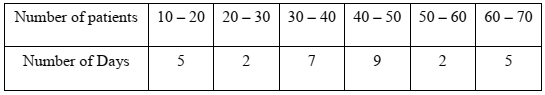Take the assumed mean as 45. Give your answer correct to 2 decimal places. 

(b) Using properties of proportion solve for x, given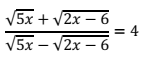(c) Sachin invests Rs. 8500 in 10%, Rs. 100 shares at Rs. 170. He sells the shares when the price of each share rises by Rs. 30. He invests the proceeds in 12% Rs. 100 shares at Rs.125. Find:

1. the sale proceeds.
2. the number of Rs. 125 shares he buys.
3. the change in his annual income. 

Question 10

(a) Use graph paper for this question.

The marks obtained by 120 students in an English test are given below: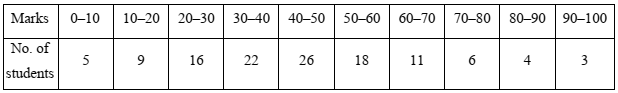Draw the ogive and hence, estimate:

1. the median marks.
2. the number of students who did not pass the test if the pass percentage was 50.
3. the upper quartile marks.

(b) A man observes the angle of elevation of the top of the tower to be 45°. He walks towards it in a horizontal line through its base. On covering 20 m the angle of elevation changes to 60°. Find the height of the tower correct to 2 significant figures. 

Question 11

(a) Using the Remainder Theorem find the remainders obtained when x3 + (kx + 8)x + k is divided by x + 1 and x – 2. Hence find k if the sum of the two remainders is 1. 

(b) The product of two consecutive natural numbers which are multiples of 3 is equal to 810. Find the two numbers. 

(c) In the given figure, ABCDE is a pentagon inscribed in a circle such that AC is a diameter and side BC//AE. If ∠BAC = 50°, find giving reasons:

1. ∠ACB
2. ∠EDC
3. ∠BEC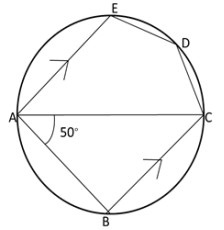Hence prove that BE is also a diameter.